# Annulus from triangle

Calculate the content of the area bounded by a circle circumscribed and a circle inscribed by a triangle with sides a = 25mm, b = 29mm, c = 36mm

Correct result:

S =  831 mm2

#### Solution:

$a=25 \ \text{mm} \ \\ b=29 \ \text{mm} \ \\ c=36 \ \text{mm} \ \\ \ \\ c_{1}=\sqrt{ a^2+b^2 }=\sqrt{ 25^2+29^2 } \doteq \sqrt{ 1466 } \ \text{mm} \doteq 38.2884 \ \text{mm} \ \\ c_{1} \ne c \ \\ \ \\ s=\dfrac{ a+b+c }{ 2 }=\dfrac{ 25+29+36 }{ 2 }=45 \ \text{mm} \ \\ A=\sqrt{ s \cdot \ (s-a) \cdot \ (s-b) \cdot \ (s-c) }=\sqrt{ 45 \cdot \ (45-25) \cdot \ (45-29) \cdot \ (45-36) }=360 \ \text{mm}^2 \ \\ \ \\ R=\dfrac{ a \cdot \ b \cdot \ c }{ 4 \cdot \ A }=\dfrac{ 25 \cdot \ 29 \cdot \ 36 }{ 4 \cdot \ 360 }=\dfrac{ 145 }{ 8 }=18.125 \ \text{mm} \ \\ \ \\ r=\dfrac{ A }{ s }=\dfrac{ 360 }{ 45 }=8 \ \text{mm} \ \\ \ \\ S_{1}=\pi \cdot \ R^2=3.1416 \cdot \ 18.125^2 \doteq 1032.0623 \ \text{mm}^2 \ \\ S_{2}=\pi \cdot \ r^2=3.1416 \cdot \ 8^2 \doteq 201.0619 \ \text{mm}^2 \ \\ \ \\ S=S_{1}-S_{2}=1032.0623-201.0619=831 \ \text{mm}^2$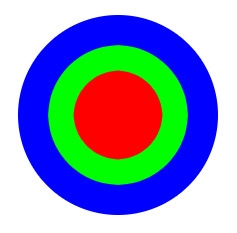Our examples were largely sent or created by pupils and students themselves. Therefore, we would be pleased if you could send us any errors you found, spelling mistakes, or rephasing the example. Thank you!

Leave us a comment of this math problem and its solution (i.e. if it is still somewhat unclear...):Be the first to comment!Tips to related online calculators
Do you want to convert area units?

#### You need to know the following knowledge to solve this word math problem:

We encourage you to watch this tutorial video on this math problem:

## Next similar math problems:Calculate the content of a regular 15-sides polygon inscribed in a circle with radius r = 4. Express the result to two decimal places.
• DodecagonCalculate the size of the smaller of the angles determined by lines A1 A4 and A2 A10 in the regular dodecagon A1A2A3. .. A12. Express the result in degrees.
• Triangle in a squareIn a square ABCD with side a = 6 cm, point E is the center of side AB and point F is the center of side BC. Calculate the size of all angles of the triangle DEF and the lengths of its sides.
• An observerAn observer standing west of the tower sees its top at an altitude angle of 45 degrees. After moving 50 meters to the south, he sees its top at an altitude angle of 30 degrees. How tall is the tower?
• Trip with compassDuring the trip, Peter went 5 km straight north from the cottage, then 12 km west and finally returned straight to the cottage. How many kilometers did Peter cover during the whole trip?
• In theIn the rectangle ABCD, the distance of its center from the line AB is 3 cm greater than from the line BC. The circumference of the rectangle is 52 cm. Calculate the contents of the rectangle. Express the result in cm2.
• Three examples per-mille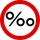A. Calculate 1.5 ‰ from 4.5 liters of blood. Express the result in milliliters. B. Calculate three ‰ from € 50,000. C. The tray they brought breakfast to the landlord's bed is made of an alloy that contains 830 ‰ of silver. How much silver did they use
• Spherical segmentCalculate the volume of a spherical segment 18 cm high. The diameter of the lower base is 80 cm, the upper base 60 cm.
• Alcohol from potatoes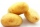In the distillery, 10 hl of alcohol can make from 8 t of potatoes. The rectangular field with dimensions of 600 m and 200 m had a yield of 20 t of potatoes per hectare. How many square meters of area are potatoes grown to obtain one liter of alcohol?
• Lookout towerHow high is the lookout tower? If each step was 3 cm lower, there would be 60 more of them on the lookout tower. If it was 3 cm higher again, it would be 40 less than it is now.
• A bottleA bottle full of cola weighs 1,320 g. If we drink three-tenths of it, it will weigh 1,008g. How much does an empty bottle weigh?
• Bike cost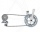The father gave his son € 100 to buy a bicycle, which was 40% of the total amount of the bicycle. How much did the bike cost?
• CoordinatesDetermine the coordinates of the vertices and the content of the parallelogram, the two sides of which lie on the lines 8x + 3y + 1 = 0, 2x + y-1 = 0 and the diagonal on the line 3x + 2y + 3 = 0
• Cuboid edgesCalculate the volume and surface of a cuboid whose edge lengths are in the ratio 2: 3: 4 and the longest edge measures 10cm.
• Tournament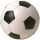How many matches will be played in a football tournament in which there are two groups of 5 teams if one match is played in groups with each other and the group winners play a match for the overall winner of the tournament?
• Finite arithmetic sequenceHow many numbers should be inserted between the numbers 1 and 25 so that all numbers create a finite arithmetic sequence and that the sum of all members of this group is 117?
• Motorcycle engineThe motorcycle engine has a constant power of 1.2 kW for 0.5 hours. How much work does the engine do? Express work in kWh and Ws units.